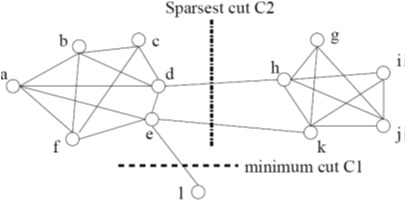# Current Slide

Small screen detected. You are viewing the mobile version of SlideWiki. If you wish to edit slides you will need to use a larger device.

### Graph Clustering: Sparsest Cut

• G = (V,E). The cut set of a cut is the set of edges {(u, v) ∈ E | u ∈ S, v ∈ T } and S and T are in two partitions• Size of the cut: # of edges in the cut set
• Min-cut (e.g., C1) is not a good partition
• A better measure: Sparsity:

φ=the size of the cut / min{|S|,|T|}

• A cut is sparsest if its sparsity is not greater than that of any other cut
• Ex. Cut C2 = ({a, b, c, d, e, f, l}, {g, h, i, j, k}) is the sparsest cut
• For k clusters, the modularity o a clustering assesses the quality of the clustering:

$Q=\sum_{i=1}^{k}(\frac{l_{i}}{|E|}-(\frac{d_{i}}{2|E|})^{2})$

li: # edges between vertices in the i-th cluster

di: the sum of the degrees of the vertices in the i-th cluster

• The modularity of a clustering of a graph is the difference between the fraction of all edges that fall into individual clusters and the fraction that would do so if the graph vertices were randomly connected
• The optimal clustering of graphs maximizes the modularitySpeaker notes:

## Content Tools

### Sources

There are currently no sources for this slide.